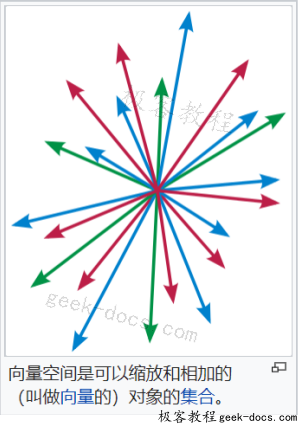# 向量空间## 公理化定义

• 向量加法 + : V × V → V，把V中的两个元素 u 和 v 映射到V中另一个元素，记作 u + v；
• 标量乘法 · : F × V → V，把F中的一个元素 a 和 V 中的一个元素u变为V中的另一个元素，记作 a ·u。

V中的元素称为向量，相对地，F中的元素称为标量。

### 基本性质

• 零向量0是唯一的；
• 对任意a ∈ F，a · 0 = 0；
• 对任意u ∈ V，0=0 ·u （0是F的加法单位元）。
• 如果a ·u = 0，则要么a = 0，要么u = 0。
• 向量加法的逆向量v是唯一的，记作− v。u + (− v)也可以写成u − v，两者都是标准的。
• 对任意u ∈ V， − u=−1 ·u .
• 对任意a ∈ F以及u ∈ V， (−a) ·u= −(a ·u) = a · (− u).
例子
对一般域F，V记为F-向量空间。若F是实数域ℝ，则V称为实数向量空间；若F是复数域ℂ，则V称为复数向量空间；若F是有限域，则V称为有限域向量空间

• u + (v + w) = (u + v) + w，
• v + w = w + v，
• 零元存在：零元0满足：对任何的向量元素v，v + 0 = v，
• 逆元素存在：对任何的向量元素v，它的相反数w = −v就满足v + w = 0。
• 标量乘法对向量加法满足分配律：a(v + w) = a v + a w.
• 向量乘法对标量加法满足分配律：(a + b)v = a v + b v.
• 标量乘法与标量的域乘法相容：a(bv) =(ab)v。
• 标量乘法有单位元：ℝ中的乘法单位元，也就是实数“1”满足：对任意实数v，1v = v。

\forall \lambda \in {\mathbb {R}},\,v=(a_{1},a_{2},\cdots ,a_{n})\in {\mathbb {R}}^{n},\,w=(b_{1},b_{2},\cdots ,b_{n})\in {\mathbb {R}}^{n}

v+w=(a_{1},a_{2},\cdots ,a_{n})+(b_{1},b_{2},\cdots ,b_{n})=(a_{1}+b_{1},a_{2}+b_{2},\cdots ,a_{n}+b_{n})

\lambda v=\lambda (a_{1},a_{2},\cdots ,a_{n})=(\lambda a_{1},\lambda a_{2},\cdots ,\lambda a_{n})

## 方程组与向量空间

3x+2y-z=0 \\ x+5y+2z=0 \\ 如果(x_{1},y_{1},z_{1})和(x_{2},y_{2},z_{2})都是解，那么可以验证它们的“和”(x_{1}+x_{2},y_{1}+y_{2},z_{1}+z_{2})也是一组解，因为：

3(x_{1}+x_{2})+2(y_{1}+y_{2})-(z_{1}+z_{2})=(3x_{1}+2y_{1}-z_{1})+(3x_{2}+2y_{2}-z_{2})=0 \\ (x_{1}+x_{2})+5(y_{1}+y_{2})+2(z_{1}+z_{2})=(x_{1}+5y_{1}+2z_{1})+(x_{2}+5y_{2}+2z_{2})=0

f”+4xf’+\cos(x)f=0

## 子空间基底

v=\lambda_{1}e_{1}+\lambda_{2}e_{2}+\cdots +\lambda_{n}e_{n}+\cdots

v=\lambda_{1}e_{1}+\lambda_{2}e_{2}+\cdots +\lambda_{n}e_{n}

e_{1}=(1,0,\cdots ,0) \\ e_{2}=(0,1,\cdots ,0) \\ e_{n}=(0,0,\cdots ,1)

## 线性映射

f:V\rightarrow W
\forall a\in F,u,v\in V,f(u+v)=f(u)+f(v),f(a\cdot v)=a\cdot f(v)

g\circ f(x)=x,f\circ g(y)=y

• 回顶